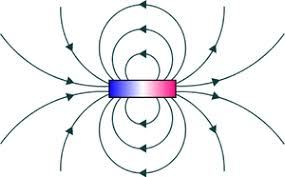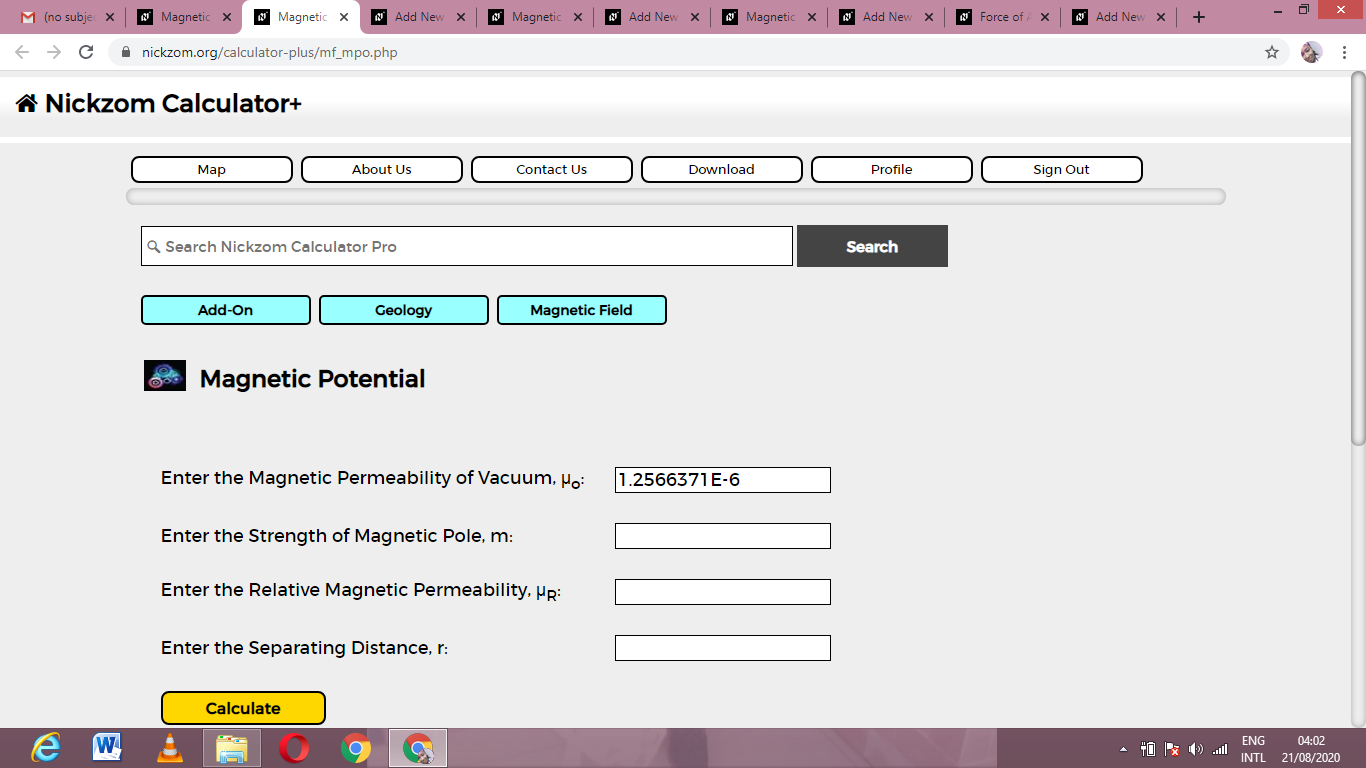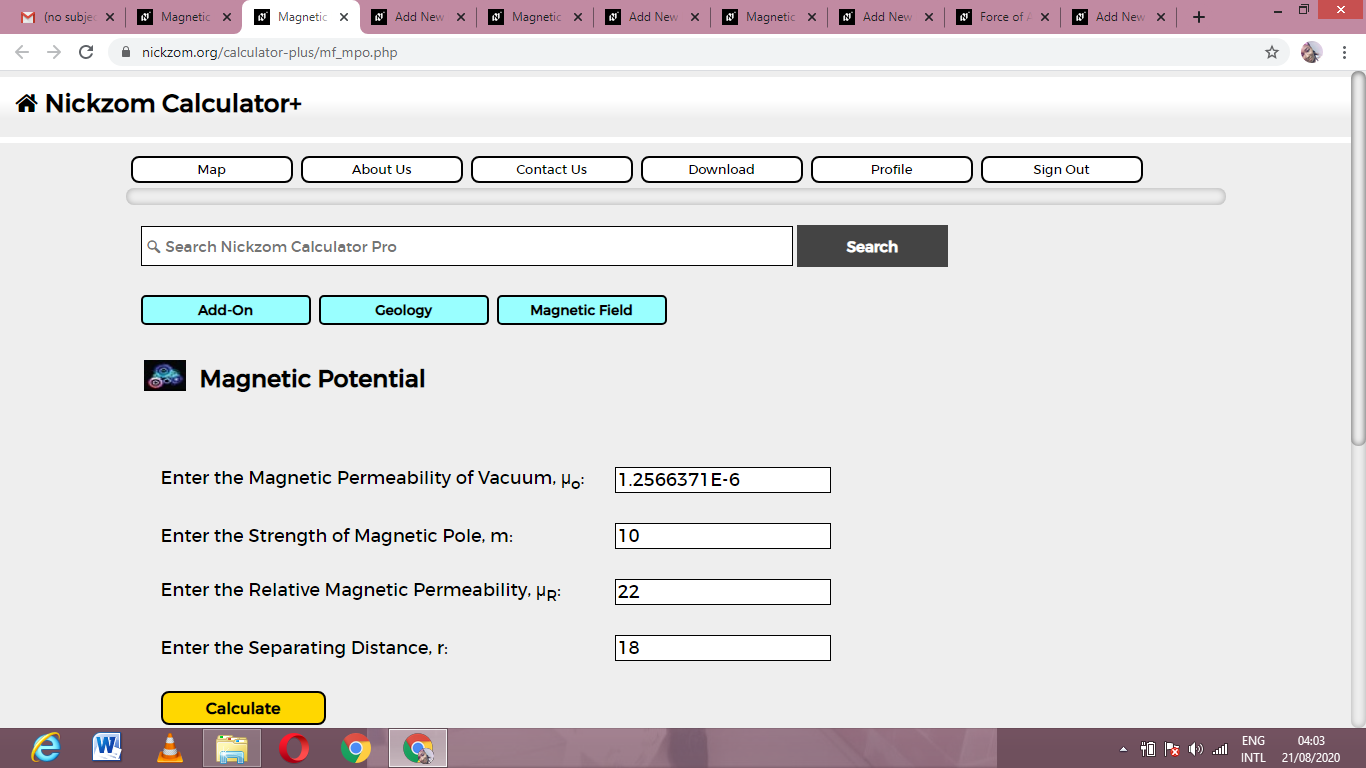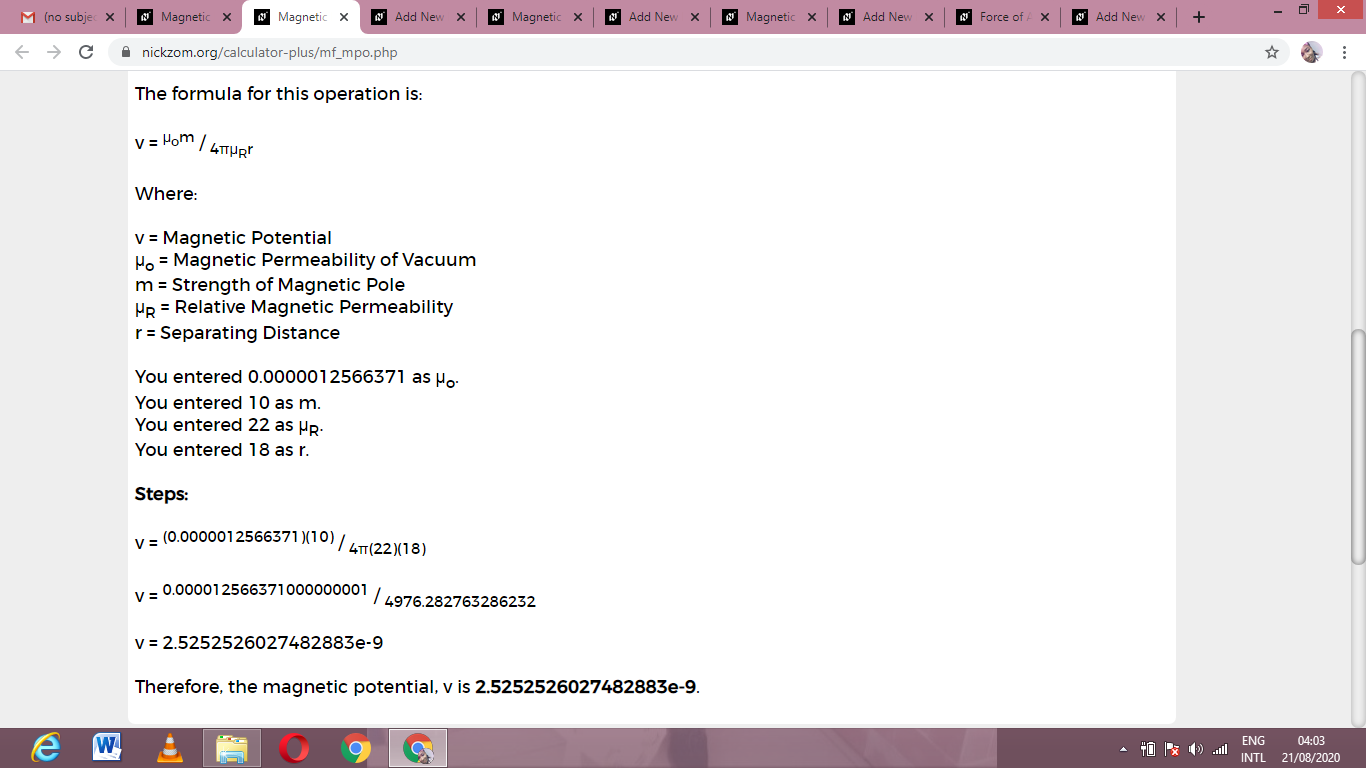# How to Calculate and Solve for Magnetic Potential | Magnetic FieldThe image above represents magnetic potential.

To compute for magnetic potential, four essential parameters are needed and these parameters are Magnetic Permeability of Vacuum (μo), Strength of Magnetic Pole (m), Relative Magnetic Permeability (μRand Seperating Distance (r).

The formula for calculating magnetic potential:

v = μom / 4πμRr

Where:

v = Magnetic Potential
μo = Magnetic Permeability of Vacuum
m = Strength of Magnetic Pole
μR = Relative Magnetic Permeability
r = Separating Distance

Let’s solve an example;
Find the magnetic potential when the magnetic permeability of vaccum is 1.2566371E-6, the strength of magnetic pole is 10, the relative magnetic permeability is 22 and seperating distance is 18.

This implies that;

μo = Magnetic Permeability of Vacuum = 1.2566371E-6
m = Strength of Magnetic Pole = 10
μR = Relative Magnetic Permeability = 22
r = Separating Distance = 18

v = μom / 4πμRr
v = (0.0000012566371)(10) / 4π(22)(18)
v = 0.000012566371000000001 / 4976.282
v = 2.52e-9

Therefore, the magnetic potential is 2.52e-9.

Nickzom Calculator – The Calculator Encyclopedia is capable of calculating the magnetic potential.

To get the answer and workings of the magnetic potential using the Nickzom Calculator – The Calculator Encyclopedia. First, you need to obtain the app.

You can get this app via any of these means:

To get access to the professional version via web, you need to register and subscribe for NGN 2,000 per annum to have utter access to all functionalities.
You can also try the demo version via https://www.nickzom.org/calculator

Apple (Paid) – https://itunes.apple.com/us/app/nickzom-calculator/id1331162702?mt=8
Once, you have obtained the calculator encyclopedia app, proceed to the Calculator Map, then click on Geology under Add-on.Now, Click on Magnetic Field under GeologyNow, Click on Magnetic Potential under Magnetic FieldThe screenshot below displays the page or activity to enter your values, to get the answer for the magnetic potential according to the respective parameters which are the Magnetic Permeability of Vacuum (μo), Strength of Magnetic Pole (m), Relative Magnetic Permeability (μRand Seperating Distance (r).Now, enter the values appropriately and accordingly for the parameters as required by the Magnetic Permeability of Vacuum (μo) is 1.2566371E-6, Strength of Magnetic Pole (m) is 10, Relative Magnetic Permeability (μRis 22 and Seperating Distance (r) is 18.Finally, Click on CalculateAs you can see from the screenshot above, Nickzom Calculator– The Calculator Encyclopedia solves for the magnetic potential and presents the formula, workings and steps too.